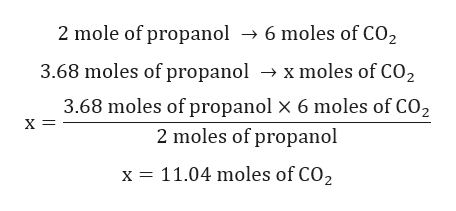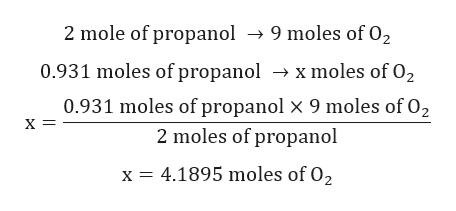# Consider the combustion of an alcohol:C3H8O(l) + O2(g) → CO2(g) + H2O(g)a. Balance the chemical equationb. How many moles of CO2 can be formed from 3.68moles of the alcohol?c. How many moles of O2 are required to react with 0.931moles of the alcohol?d. What is the maximum number of moles of CO2 that can be formed from a mixture of 1.81moles of the alcohol and 7.43moles of O2

Question
286 views

Consider the combustion of an alcohol:
C3H8O(l) + O2(g) → CO2(g) + H2O(g)
a. Balance the chemical equation
b. How many moles of CO2 can be formed from 3.68moles of the alcohol?
c. How many moles of O2 are required to react with 0.931moles of the alcohol?
d. What is the maximum number of moles of CO2 that can be formed from a mixture of 1.81moles
of the alcohol and 7.43moles of O2

check_circle

Step 1

Balanced chemical reaction is given by:

Step 2

Calculation for moles of CO2:help_outlineImage Transcriptionclose2 mole of propanol -> 6 moles of CO2 3.68 moles of propanol - x moles of CO2 3.68 moles of propanol x 6 moles of CO2 X 2 moles of propanol x 11.04 moles of CO2 fullscreen
Step 3

Calculation for moles of O2 required to wi...help_outlineImage Transcriptionclose2 mole of propanol -»9 moles of 02 0.931 moles of propanol -> xmoles of 02 0.931 moles of propanol x 9 moles of 02 х 2 moles of propanol X 4.1895 moles of 02 fullscreen

### Want to see the full answer?

See Solution

#### Want to see this answer and more?

Solutions are written by subject experts who are available 24/7. Questions are typically answered within 1 hour.*

See Solution
*Response times may vary by subject and question.
Tagged in

### General Chemistry